# What Is The Relation Between Artificial And Biological Neuron?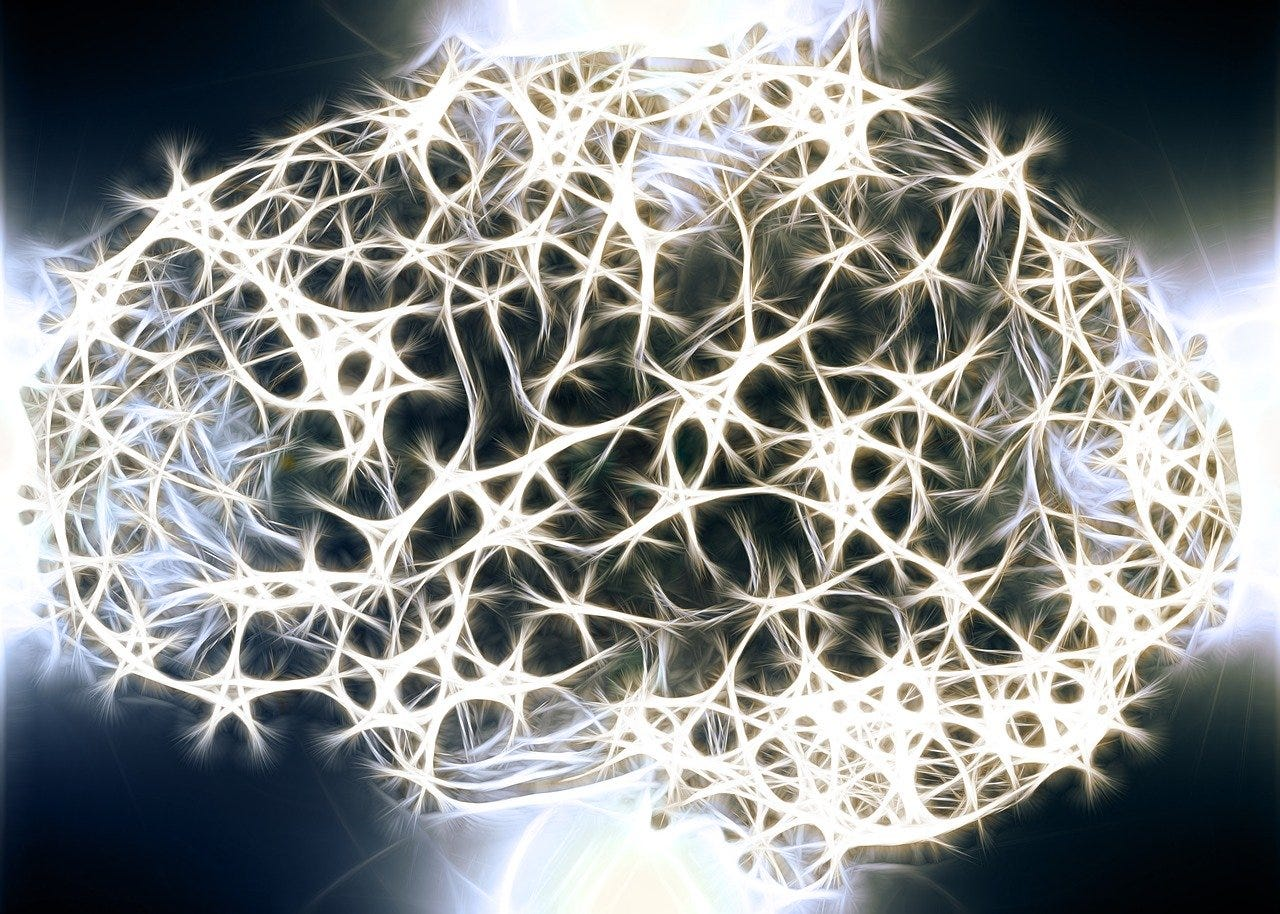Image by Gerd Altmann from Pixabay

We have heard of the latest advancements in the field of deep learning due to the usage of different neural networks. Most of these achievements are simply astonishing and I find myself amazed after reading every new article on the advancements in this field almost every week. At the most basic level, all such neural networks are made up of artificial neurons that try to mimic the working of biological neurons. I had a curiosity about understanding how these artificial neurons compare to the structure of biological neurons in our brains and if possibly this could lead to a way to improve neural networks further. So if you are curious about this topic too, then let’s embark on a short 5-minute journey to understand this topic in detail…

First, let’s understand how biological neurons work inside our brains…

# Biological Neurons

Neurons are the basic functional units of the nervous system, and they generate electrical signals called action potentials, which allows them to quickly transmit information over long distances.
Almost all the neurons have three basic functions essential for the normal functioning of all the cells in the body.

These are to:
1. Receive signals (or information) from outside.
2. Process the incoming signals and determine whether or not the information should be passed along.
3. Communicate signals to target cells which might be other neurons or muscles or glands.

Now let us understand the of a neuron to get a deeper insight into how they actually work…

A biological neuron is mainly composed of 3 main parts and an external part called synapse:-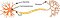Image source: Wikimedia Commons
1. Dendrite

Dendrites are responsible for getting incoming signals from outside

2. Soma

Soma is the cell body responsible for the processing of input signals and deciding whether a neuron should fire an output signal

3. Axon

Axon is responsible for getting processed signals from neuron to relevant cells

4. Synapse

Synapse is the connection between an axon and other neuron dendrites

## Working of the parts

The task of receiving the incoming information is done by dendrites, and processing generally takes place in the cell body. Incoming signals can be either excitatory — which means they tend to make the neuron fire (generate an electrical impulse) — or inhibitory — which means that they tend to keep the neuron from firing.

Most neurons receive many input signals throughout their dendritic trees. A single neuron may have more than one set of dendrites and may receive many thousands of input signals. Whether or not a neuron is excited into firing an impulse depends on the sum of all of the excitatory and inhibitory signals it receives. The processing of this information happens in soma which is neuron cell body. If the neuron does end up firing, the nerve impulse, or action potential, is conducted down the axon.

Towards its end, the axon splits up into many branches and develops bulbous swellings known as axon terminals (or nerve terminals). These axon terminals make connections on target cells.

# Artificial Neurons

Artificial neuron also known as perceptron is the basic unit of the neural network. In simple terms, it is a mathematical function based on a model of biological neurons. It can also be seen as a simple logic gate with binary outputs. They are sometimes also called perceptrons.

Each artificial neuron has the following main functions:

1. Takes inputs from the input layer
2. Weighs them separately and sums them up
3. Pass this sum through a nonlinear function to produce output.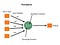Basic perceptron diagram

The perceptron(neuron) consists of 4 parts:

1. Input values or One input layer
We pass input values to a neuron using this layer. It might be something as simple as a collection of array values. It is similar to a dendrite in biological neurons.
2. Weights and Bias
Weights are a collection of array values which are multiplied to the respective input values. We then take a sum of all these multiplied values which is called a weighted sum. Next, we add a bias value to the weighted sum to get final value for prediction by our neuron.
3. Activation Function
Activation Function decides whether or not a neuron is fired. It decides which of the two output values should be generated by the neuron.
4. Output Layer
Output layer gives the final output of a neuron which can then be passed to other neurons in the network or taken as the final output value.

Now, all the above concepts might seem like too much theoretical knowledge without any practical insights, so let’s understand the working of an artificial neuron with an example.

Consider a neuron with two inputs (x1,x2) as shown below: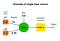Single-layer neuron example
1. The values of the two inputs(x1,x2) are 0.8 and 1.2
2. We have a set of weights (1.0,0.75) corresponding to the two inputs
3. Then we have a bias with value 0.5 which needs to be added to the sum

The input to activation function is then calculated using the formula:-Calculation of `C` value

Now the combination(C) can be fed to the activation function. Let us first understand the logic of Rectified linear (ReLU) activation function which we are currently using in our example: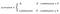ReLU activation function

In our case, the combination value we got was 2.2 which is greater than 0 so the output value of our activation function will be 2.2.

This will be the final output value of our single layer neuron.

# Biological Neuron vs. Artificial Neuron

Since we have learnt a bit about both biological and artificial neurons we can now draw comparisons between both as follows: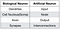Similarities between biological and artificial neuron

Revision of concepts

Let’s have a short recap of the concepts to remember them for a longer time…

• A neuron is a mathematical function modelled on the working of biological neurons
• It is an elementary unit in an artificial neural network
• Inputs are first multiplied by weights, then summed and passed through a nonlinear function to produce output

And that brings us to the end of our short 5-minute journey…My inbox is always open to solve doubts or taking suggestions on Linkedin or Twitter.

References:

1. Khan Academy Series on Biological neurons
2. Simplilearn article on perceptrons
3. Neural Designer article on the example of perceptrons

Blogger,web and hybrid applications developer

## More from Saurabh Mhatre

Blogger,web and hybrid applications developer